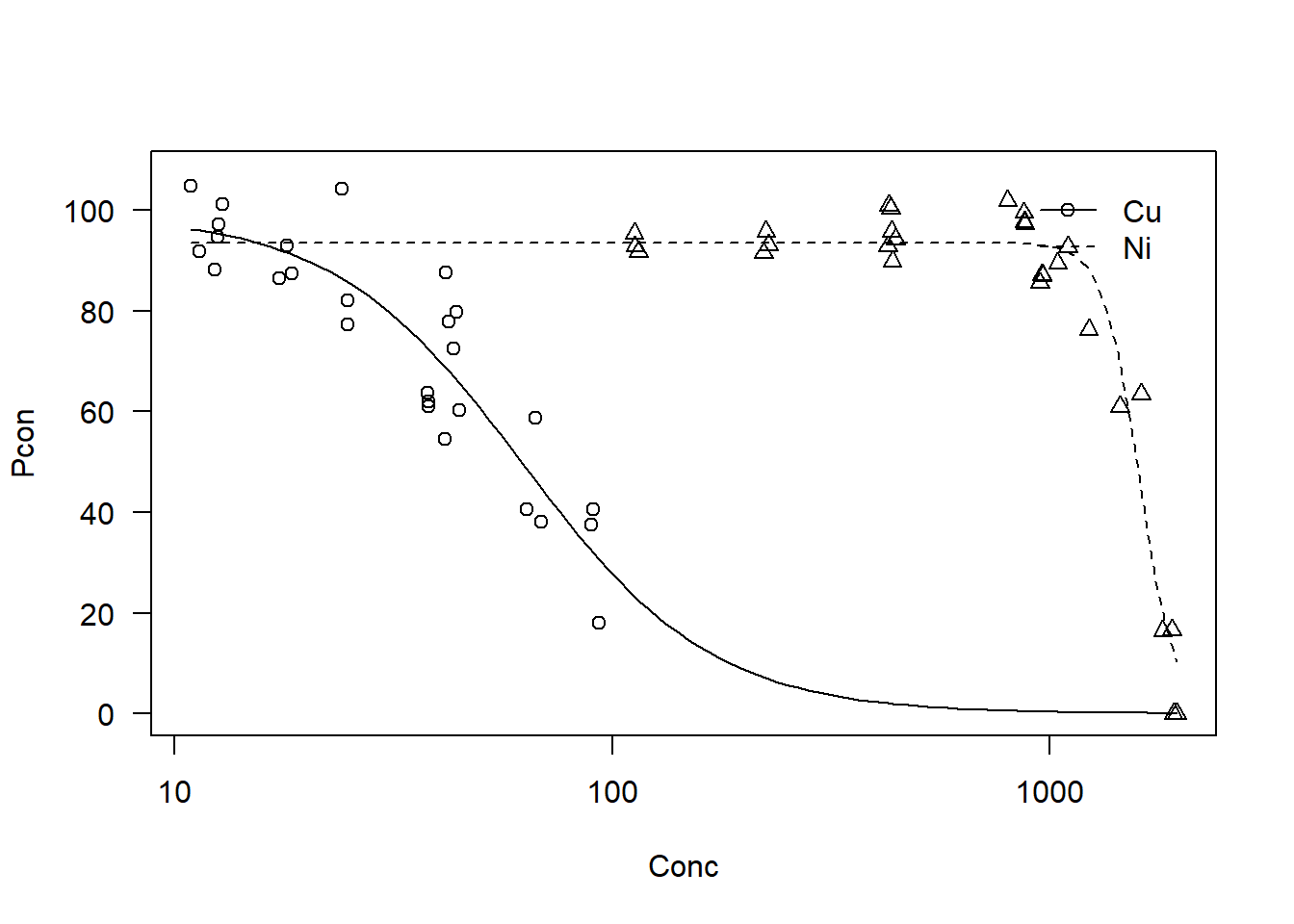The data comes from https://data.aad.gov.au/metadata/records/AAS_4326_microalga_toxicity which is published in this paper https://doi.org/10.1016/j.envpol.2017.05.034.

The approach is outlined by Ritz et al (2016) in their R ‘drc’ package, check out its description here. My understanding is that comped function is based on the Wheeler et al (2006) ratio test, while the EDcomp function comes from a Ritz et al (2006) paper

Look at the structure, it contains 3 columns: Metal (with 2 factor levels), the numeric Conc, and the numeric Pcon. Pcon is our response (Growth Rate as percent of control), Conc is the measured concentration of Metal, which has Cu and Ni data.

``library(drc)``
``````dat<-read.table("EC50comparison.csv", header=T, sep=",")
``````##   Metal     Conc      Pcon
## 1    Cu 12.61106  97.17794
## 2    Cu 12.54240  94.60096
## 3    Cu 12.84345 101.18122
## 4    Cu 43.45770  72.50561
## 5    Cu 42.39194  77.84305
## 6    Cu 44.07704  79.75710``````

### Run drm model

I’m going to run a 3 parameter log logistic model on the data sets. You can do both at once by telling the drm function to look at factor levels in the column “Metal”

``````models<- drm(Pcon~Conc, Metal, data = dat, fct = LL.3())
plot(models)``````Looks like there is a clear difference, and the EC50 confidence intervals don’t overlap:

``ED(models, c(50), interval="delta")``
``````##
## Estimated effective doses
##
##          Estimate Std. Error     Lower     Upper
## e:Cu:50   62.4714     3.9288   54.6498   70.2930
## e:Ni:50 1600.2703    60.0883 1480.6436 1719.8970``````

### Significance testing between the two EC50s

Because we have the full dataset that generated the code, its recommended we use the EDcomp function, which returns a highly significant p value

``EDcomp(models, c(50,50))``
``````##
## Estimated ratios of effect doses
##
##                 Estimate   Std. Error      t-value      p-value
## Cu/Ni:50/50   3.9038e-02   2.8594e-03  -3.3607e+02  2.3533e-125``````

Alternatively you can use the comped function if you have the EC50 values and standard errors.

``comped(c(61.98, 1600), c(2.4589,52.1428),log= FALSE, level = 0.95, operator = c("/"))``
``````##
## Estimated ratio of effective doses
##
##       Estimate Std. Error     Lower  Upper
## [1,] 0.0387375  0.0019888 0.0348394 0.0426``````
``#If the confidence interval (range of lower and upper) contains 1, then it's not considered significant. In this case it doesn't, so there is a significant difference. ``